# The HPMIXED Procedure

### Mixed Model with Large Number of Fixed and Random Effects

In animal breeding, it is common to model genetic and environmental effects with a random effect for the animal. When there are many animals being studied, this can lead to very large mixed model equations to be solved. In this example we present an analysis of simulated data with this structure.

Suppose you have 3000 animals from five different genetic species raised on 100 different farms. The following DATA step simulates 40000 observations of milk yield (Yield) from a linear mixed model with variables Species and Farm in the fixed-effect model and Animal as a random effect. The random effect due to Animal is simulated with a variance of 4.0, while the residual error variance is 8.0. These variance component values reflect the fact that variation in milk yield is typically genetically controlled to be no more than 33% (4/(4+8)).

data Sim;
keep Species Farm Animal Yield;
array AnimalEffect{3000};
array AnimalFarm{3000};
array AnimalSpecies{3000};
do i = 1 to dim(AnimalEffect);
AnimalEffect{i}  = sqrt(4.0)*rannor(12345);
AnimalFarm{i}    = 1 + int(100*ranuni(12345));
AnimalSpecies{i} = 1 + int(5*ranuni(12345));
end;
do i = 1 to 40000;
Animal  = 1 + int(3000*ranuni(12345));
Species = AnimalSpecies{Animal};
Farm    = AnimalFarm{Animal};
Yield   = 1 + Species + Farm/10 + AnimalEffect{Animal}
+ sqrt(8.0)*rannor(12345);
output;
end;
run;


A simple linear mixed model analysis is performed by using the following SAS statements:

proc hpmixed data=Sim;
class Species Farm Animal;
model Yield = Species Species*Farm;
random Animal;
test Species*Farm;
contrast 'Species1 = Species2 = Species3'
Species 1 0 -1,
Species 0 1 -1;
run;


Selected results from the preceding SAS statements are shown in Figure 49.1 through Figure 49.4.

The "Class Level Information" table in Figure 49.1 shows that the three model effects have 5, 100, and 3000 levels, respectively. Only a portion of the levels are displayed by default. The "Dimensions" table shows that the model contains a single G-side covariance parameter and a single R-side covariance parameter. R-side covariance parameters are those associated with the covariance matrixin the conditional distribution, given the random effects. In the case of the HPMIXED procedure this matrix is simply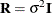and the single R-side covariance parameter corresponds to the residual variance. The G-side parameter is the variance of the random Animal effect; thematrix is a diagonal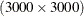matrix with the common variance on the diagonal.

Figure 49.1: Class Levels and Dimensions

The HPMIXED Procedure

Class Level Information
Class Levels Values
Species 5 1 2 3 4 5
Farm 100 1 2 3 4 5 6 7 8 9 10 11 12 13 14 15 16 17 18 19 20 ...
Animal 3000 1 2 3 4 5 6 7 8 9 10 11 12 13 14 15 16 17 18 19 20 ...

Dimensions
G-side Cov. Parameters 1
R-side Cov. Parameters 1
Columns in X 506
Columns in Z 3000
Subjects (Blocks in V) 1

Taking into account the intercept as well as the number of levels of the Species and Species*Farm effects, thematrix for this problem has 506 columns, so that the mixed model equations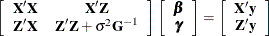have 3506 rows and columns. This is a substantial computational problem: simply storing a single copy of this matrix in dense format requires nearly 50 megabytes of memory. The sparse matrix techniques of PROC HPMIXED use a small fraction of this amount of memory and a similarly small fraction of the CPU time required to solve the equations with dense techniques. For more information about sparse versus dense techniques, see the section Sparse Matrix Techniques.

Figure 49.2 displays the covariance parameter estimates at convergence of the REML algorithm. The variance component estimate for animal effect is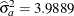and for residual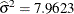. These estimates are close to the simulated values (4.0 and 8.0).

Figure 49.2: Estimates of Variance Components

Covariance Parameter
Estimates
Cov Parm Estimate
Animal 3.9889
Residual 7.9623

The TEST statement requests a Type III test of the fixed effect in the model. By default, the HPMIXED procedure does not compute Type III tests, because they can be computationally demanding. The tests of the Species*Farm effect is highly significant. That indicates animals of a genetic species perform differently in different environments.

Figure 49.3: Type III Tests of Fixed Effect

Type III Tests of Fixed Effects
Effect Num DF Den DF F Value Pr > F
Species*Farm 495 39500 11.72 <.0001

You can use the CONTRAST or ESTIMATE statement to test custom linear hypotheses involving the fixed and/or random effects. The CONTRAST statement in the preceding program tests the null hypothesis that there are no differences among the first three genetic species. Results from this analysis are shown in Figure 49.4. The small p-value indicates that there are significant differences among the first three genetics species.

Figure 49.4: Result of CONTRAST Statement

Contrasts
Label Num DF Den DF F Value Pr > F
Species1 = Species2 = Species3 2 39500 92.93 <.0001